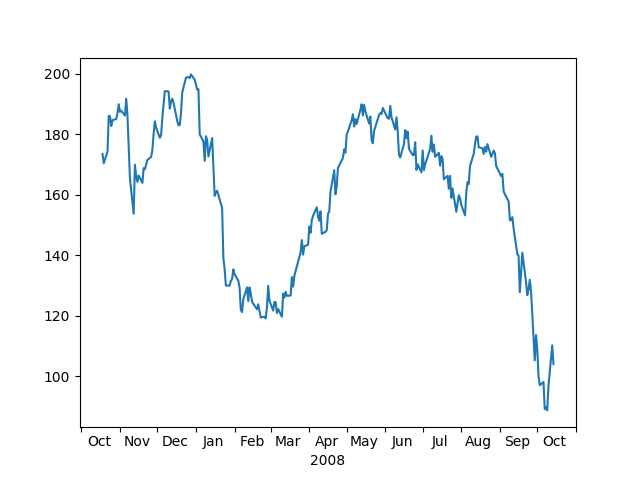Travis-CI:# Centered Ticklabels¶

sometimes it is nice to have ticklabels centered. Matplotlib currently associates a label with a tick, and the label can be aligned ‘center’, ‘left’, or ‘right’ using the horizontal alignment property:

```ax.xaxis.set_tick_params(horizontalalignment='right')
```

but this doesn’t help center the label between ticks. One solution is to “fake it”. Use the minor ticks to place a tick centered between the major ticks. Here is an example that labels the months, centered between the ticks```import numpy as np
import matplotlib.cbook as cbook
import matplotlib.dates as dates
import matplotlib.ticker as ticker
import matplotlib.pyplot as plt

# load some financial data; apple's stock price
with cbook.get_sample_data('aapl.npz') as fh:
r = r[-250:]  # get the last 250 days
# Matplotlib works better with datetime.datetime than np.datetime64, but the
# latter is more portable.
date = r.date.astype('O')

fig, ax = plt.subplots()

ax.xaxis.set_major_locator(dates.MonthLocator())
ax.xaxis.set_minor_locator(dates.MonthLocator(bymonthday=15))

ax.xaxis.set_major_formatter(ticker.NullFormatter())
ax.xaxis.set_minor_formatter(dates.DateFormatter('%b'))

for tick in ax.xaxis.get_minor_ticks():
tick.tick1line.set_markersize(0)
tick.tick2line.set_markersize(0)
tick.label1.set_horizontalalignment('center')

imid = len(r) // 2
ax.set_xlabel(str(date[imid].year))
plt.show()
```

Total running time of the script: ( 0 minutes 0.025 seconds)

Gallery generated by Sphinx-Gallery